# Xcos vs. Simulink® – Discrete time library conversion

In the table below you can find the equivalent between the Simulink® and Xcos blocks for Discrete time library/palette. The block which are not present in both libraries are omitted from the table.

This conversion table can be used in case you need to convert a Simulink® model to an Xcos block diagram model or vice-versa.

 Simulink Discrete time library Xcos Discrete time palette Xcos block description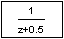Discrete Transfer Function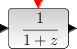Discrete transfer function This block realizes a SISO linear system represented by its rational transfer function (in the symbolic variable z). The rational function must be proper.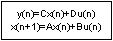Discrete State Space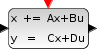Discrete state-space system This block realizes a discrete-time linear state-space system. The system is defined by the matrices and the initial state . The dimensions must be compatible. At the arrival of an input event on the unique input event port, the state is updated.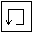Memory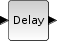Delay This compiled super-block implements a discretized delay. It is built with a shift register and a clock. The value of the delay is given by the discretization time step multiplied by the number-1 of state of the register.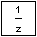Unit Delay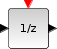Delay operator The Unit Delay block delays its input by the specified sample period. This block is equivalent to the 1/z discrete-time operator. The block accepts one input and generates one output, which can be either both scalar or both vector. If the input is a vector, all elements of the vector are delayed by the same sample period.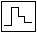Zero-order Hold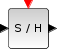Sample and hold Each time an input event is received block copy its input on the output and hold it until input event. For periodic Sample and hold, event input must be generated by a Clock.

Let’s look at a simple example in which we create a Simulink® block diagram model, simulate it and plot the results. We’ll recreate the equivalent model in Xcos and check if the results are the same.

### Simulink® block diagram model

The purpose of the model is only to use some of the Discrete time Simulink® blocks which have an equivalent in the Xcos palette.

The main parameters of each block are displayed under the name. The same parameters are going to be used for the Xcos block diagram.

By running the Simulink® model above, we get the following plot window:

### Xcos block diagram model

We follow the same approach for the Xcos block diagram model. Using equivalent blocks (see table above), we recreate the same functionality of the Simulink model.

The block parameters have the same values as those in the Simulink block diagram.

After running the Xcos model for `10 s`, we get the following graphical window:

As you can see, the outputs of the Xcos block diagram model are identical with the Simulink results (except one step delay extra on the second plot). This proves that we can use Xcos as an alternative to Simulink, when we want to simulate and analyze discrete time models.

For any questions, observations and queries regarding this article, use the comment form below.

Don’t forget to Like, Share and Subscribe!

#### Ad Blocker Detected

Dear user, Our website provides free and high quality content by displaying ads to our visitors. Please support us by disabling your Ad blocker for our site. Thank you!